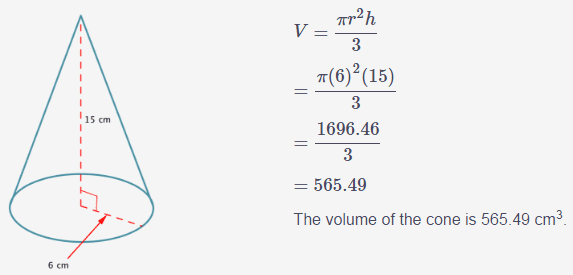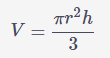# Need help understanding volumes of cones

## Homework Statement

This was a example but I didn't quite understand it, I don't know how they ended up with 565.49 every time I try I get some random other number.## Homework Equations## The Attempt at a Solution

So this is how i do it.Pi (6) 2 (15)/3

So first I multiply 3.14 (pi) by 6. so 3.14 x 6 = 18.84.
Now 18.84 to the power of 2 is equal to 354.9456
354.9456 x 15 = 5324.184
5324.184 / 3 = 1774.728.

I know im wrong but i just want to know how come when i try to calculate the volume it doesn't work???

SammyS
Staff Emeritus
Homework Helper
Gold Member

## Homework Statement

This was a example but I didn't quite understand it, I don't know how they ended up with 565.49 every time I try I get some random other number.
View attachment 100590

## Homework Equations

View attachment 100591

## The Attempt at a Solution

So this is how i do it.

View attachment 100592
Pi (6) 2 (15)/3

So first I multiply 3.14 (pi) by 6. so 3.14 x 6 = 18.84.
Now 18.84 to the power of 2 is equal to 354.9456
354.9456 x 15 = 5324.184
5324.184 / 3 = 1774.728.

I know im wrong but i just want to know how come when i try to calculate the volume it doesn't work???
You are squaring π as well as squaring r.

Review ' Order of Operations '.

ok i get it now, thx alot.